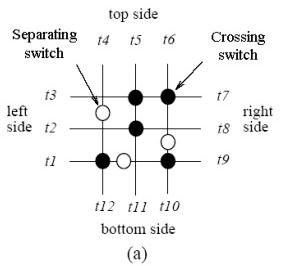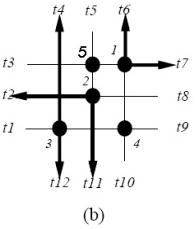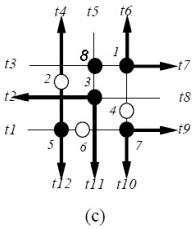# 1 figure a below shows a switch matrix with three horizontal and three vertical trac 5346586

1. Figure (a) below shows a switch matrix with three horizontal and three vertical tracks. There are two types of switches in the switch matrix, crossing switches and separating switches. (The crossing switches are represented by solid circles and the separating switches by hollow circles.) If a crossing switch at the intersection of a horizontal and a vertical tracks is “ON,” the two tracks are connected; if it is “OFF,”
the tracks are not connected and thus are electrically non-interacting. If a separating switch on a track is “OFF,” the track is split into two electrically non-interacting routing segments so that the terminals on opposite sides can be used independently; if it is “ON,” the track becomes a single electrical track.

A connection is an electrical path between two terminals on diﬀerent sides of a switch module. Assume that at most one switch can be used, i.e., programmed to be “ON,” by a connection. (Based
on this assumption, only straight connections can use separating switches. Figures (b) and (c) show some legal connections.) Let the numbers of connections required to be routed through a switch module between the top side and the right side, between the left side and the right side, and between the bottom side and the right side, be n t , n l , and n b , respectively (i.e., for this problem, we consider the nets routing through the right side only). You are asked to answer if a switch matrix can accommodate such n t , n l , and n b connections simultaneously with no connection being electrically shorted.

(a) For the switch matrix shown in Figure (b) (which has no separating switch), formulate this problem as a bipartite matching problem.
(b) For the switch matrix shown in Figure (c) (which contains separating switches), formulate this problem as a maximum ﬂow problem.